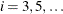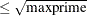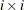### Example 5.4 Set Manipulation

This example demonstrates PROC OPTMODEL set manipulation operators. These operators are used to compute the set of primes up to a given limit. This example does not solve an optimization problem, but similar set manipulation could be used to set up an optimization model. Here are the statements:

proc optmodel;
number maxprime;  /* largest number to consider */
set composites =
union {i in 3..sqrt(maxprime) by 2} i*i..maxprime by 2*i;
set primes = {2} union (3..maxprime by 2 diff composites);
maxprime = 500;
put primes;


The set composites contains the odd composite numbers up to the value of the parameter maxprime. The even numbers are excluded here to reduce execution time and memory requirements. The UNION aggregation operation is used in the definition to combine the sets of odd multiples offor. Any composite number less than the value of the parameter maxprime has a divisor, so the range ofcan be limited. The set of multiples ofcan also be started atsince smaller multiples are found in the set of multiples for a smaller index.

You can then define the set primes. The odd primes are determined by using the DIFF operator to remove the composites from the set of odd numbers no greater than the parameter maxprime. The UNION operator adds the single even prime,, to the resulting set of primes.

The PUT statement produces the result in Output 5.4.1.

Output 5.4.1: Primes less than or equal to 500

 {2,3,5,7,11,13,17,19,23,29,31,37,41,43,47,53,59,61,67,71,73,79,83,89,97,101,103, 107,109,113,127,131,137,139,149,151,157,163,167,173,179,181,191,193,197,199,211, 223,227,229,233,239,241,251,257,263,269,271,277,281,283,293,307,311,313,317,331, 337,347,349,353,359,367,373,379,383,389,397,401,409,419,421,431,433,439,443,449, 457,461,463,467,479,487,491,499} 

Note that you were able to delay the definition of the value of the parameter maxprime until just before the PUT statement. Since the defining expressions of the SET declarations are handled symbolically, the value of maxprime is not necessary until you need the value of the set primes. Because the sets composites and primes are defined symbolically, their values reflect any changes to the parameter maxprime. You can see this update by appending the following statements to the preceding statements:

   maxprime = 50;
put primes;


The additional statements produce the results in Output 5.4.2. The value of the set primes has been recomputed to reflect the change to the parameter maxprime.

Output 5.4.2: Primes less than or equal to 50

 {2,3,5,7,11,13,17,19,23,29,31,37,41,43,47}